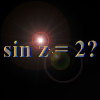#### You may also like### Big, Bigger, Biggest

Which is the biggest and which the smallest of $2000^{2002}, 2001^{2001} \text{and } 2002^{2000}$?### Complex Sine

Solve the equation sin z = 2 for complex z. You only need the formula you are given for sin z in terms of the exponential function, and to solve a quadratic equation and use the logarithmic function.### Sierpinski Triangle

What is the total area of the triangles remaining in the nth stage of constructing a Sierpinski Triangle? Work out the dimension of this fractal.

# Log Attack

##### Age 16 to 18 Challenge Level:
Case (i)

If $a = b$ the equation $a^x + b^x = 1$ where $0< a, b < 1$ and $a + b < 1$ becomes $2a^x =1$. Then $\log 2 + x \log a = 0$ so the solution can be given in three equivalent forms: $$x = {-\log 2 \over \log a} = {\log 0.5 \over \log a } = {\log 2 \over \log 1/a}.$$ Note we can use natural logarithms here or logarithms to any base.

Case (ii)

Solve $a^x + b^x = 1$, where $a=1/2$ and $b=1/4$. The equation is: $$(1/2)^x + (1/4)^x = 1,$$ Here you could substitute $y = (1/2)^x$ but Trevor multiplied by $4^x$ and used the substitution $y = 2^x$. By Trevor's method: $$4^x/2^x + 1 = 4^x$$ So $$2^x + 1 = (2^x)^2.$$ and the equation becomes: $y + 1= y^2$ or $y^2- y- 1 = 0$. Using the quadratic formula, the negative solution to the quadratic can be ignored as $2^x$ is never negative, so the solution is: $$y={1+ \sqrt 5 \over 2}$$ (note this equals the golden ratio, $\phi$). Therefore $2^x = \phi$ and so $x\ln 2 = \ln \phi$ and the solution is: $$x= {\ln \phi \over \ln 2} ={ \ln (1 + \sqrt 5)/2 \over \ln 2}$$ giving $x = 0.69424$ to 5 significant figures.